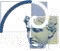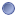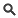### Module Detail Information

 Name: MINC MathType:Module Short URL: `http://bit.ly/1AtBg1T` Description: Minc Math allows for most common mathematical operations on minc volumes(such as addition, multiplication, thresholding, etc.). Executable: `/mincmath` Input Parameters: - Byte  - Short  - Long  - Double  - Float  - Signed  - Unsigned  - Range  - Ignore Invalid Data  - Illegal Value  - Constant  - Constant2  - Add Volumes  - Subtract by Constant  - Multiply by Constant  - Divide by Constant  - Invert Volume  - Square Root  - Square  - Absolute Value  - Maximum  - Minimum  - Exponential  - Logarithm  - Scale  - Clamp  - Segment  - Nsegment  - Percent Difference  - Equality of Volumes  - Not Equal (Volumes)  - Greater Than (volumes)  - Greater Than or Equal (volumes)  - Less Than (volumes)  - Less Than or Equal (volumes)  - And  - Or  - Not  - isNaN  - nisNaN  - Count Valid  - Overwrite (clobber)  - Do Not Overwrite (noclobber)  - Verbose  - Quiet  - Debug  - Copy Header  - Nocopy Header  - File-type  - Max Buffer Size  - Dimension  - Check Dimensions  - No-check Dimensions  - Propagate NaN  - NaN  - Zero  - Integer  - Input Mincs  - Less Than (vol & int)  - Less Than or Equal (vol & int)  - Equality of Volume and Constant  - Divide Volumes  - Greater Than (vol & int)  - Subtract Volumes  - Invert by Constant  - Greater Than or Equal (vol & int)  - Add by Constant  - Multiply Volumes  - Not Equal (Vol & Int) Output Parameters: - Output Minc File size: 48.75 KBView SourceDownloadOpen